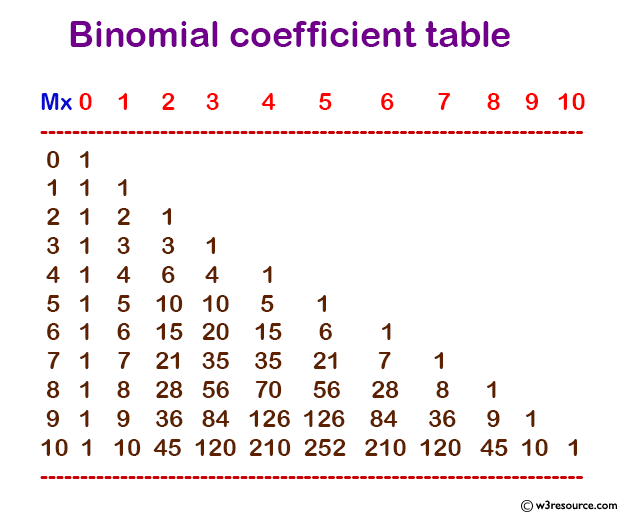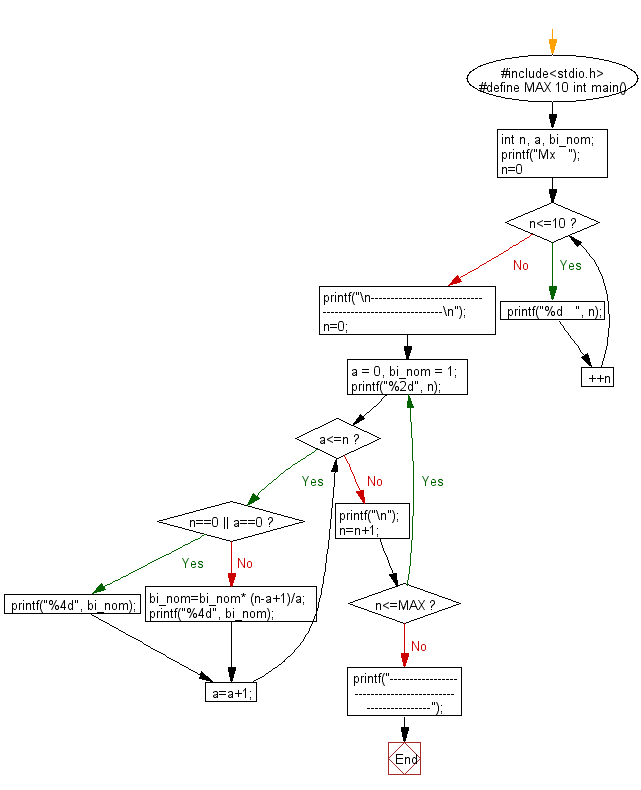﻿ C Program: Print a binomial coefficient table - w3resource

# C Exercises: Print a binomial coefficient table

## C Basic Declarations and Expressions: Exercise-69 with Solution

Write a C program to print a binomial coefficient table.

Pictorial Presentation:Sample Solution:

C Code:

``````#include<stdio.h>
#define MAX 10

int main() {
int n, a, bi_nom;

printf("Mx   ");
for (n = 0; n <= 10; ++n)
printf("%d   ", n);
printf("\n----------------------------------------------------------\n");

n = 0;

do {
a = 0, bi_nom = 1;
printf("%2d", n);

while (a <= n) {
if (n == 0 || a == 0)
printf("%4d", bi_nom);
else {
bi_nom = bi_nom * (n - a + 1) / a;
printf("%4d", bi_nom);
}
a = a + 1;
}

printf("\n");
n = n + 1;
} while (n <= MAX);

// Print footer
printf("----------------------------------------------------------");

return 0;
}
``````

Sample Output:

```Mx   0   1   2   3   4   5   6   7   8   9   10
----------------------------------------------------------
0   1
1   1   1
2   1   2   1
3   1   3   3   1
4   1   4   6   4   1
5   1   5  10  10   5   1
6   1   6  15  20  15   6   1
7   1   7  21  35  35  21   7   1
8   1   8  28  56  70  56  28   8   1
9   1   9  36  84 126 126  84  36   9   1
10   1  10  45 120 210 252 210 120  45  10   1
----------------------------------------------------------
```

Flowchart:C programming Code Editor:

What is the difficulty level of this exercise?

Test your Programming skills with w3resource's quiz.

﻿